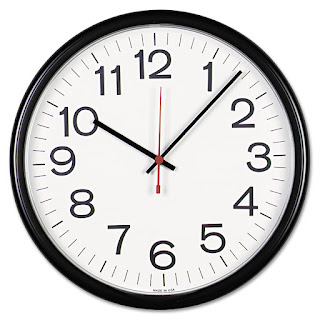## Posts

Showing posts from January, 2019

### Problem #13 - a circular trainPt En < change language This is that type of problem that can be phrased as a riddle that you can tell everyone. Of course some people will try to think it through while other people will just ask you for the solution. Problem statement : suppose you are in a circular train, i.e. a train where the first carriage is connected to the last carriage. Suppose, furthermore, that all carriages are identical and that you have no way to tell them apart. Additionally, each carriage has a light bulb that you can turn on and off to your own liking. If you are put inside such a train, how can you find how many carriages the train has, assuming that in the beginning the light bulbs have an unknown state? To sum up, the main characteristics of this riddle are: the train is circular, hence you can go forward indefinitely; the same backwards; all train carriages are exactly identical, so you can't tell them apart; each carriage has a light bulb that you can turn on/off;

### Introduction to the Hill CipherPt En < change language The Hill cipher is a very simple cipher that works by using modular arithmetic and matrices. In a nutshell, your key is a matrix in some \$\mathbb{Z}_m\$ and you encrypt messages by breaking them up into pieces and then multiplying the pieces by the key matrix. That's it. I will be giving a workshop on this subject in a near future, and so I decided to write a Python notebook with a brief explanation of how the Hill cipher works, as well as providing an implementation of said cipher. The notebook can be downloaded and read here . I will be glad if you leave any suggestions/comments in the section below! A cifra de Hill é uma cifra simples que faz uso de noções de aritmética modular e de álgebra linear (mais concretamente, matrizes). Em duas frases, a cifra de Hill tem como chave uma matriz num dado \$\mathbb{Z}_m\$ e o modo como encripta mensagens é partindo-a em bocados com o mesmo tamanho e depois multiplicando a matriz chave por

### Problem #12 - it is just a matter of timePt En < change language Today's problem is really simple! But when you know the solution, you can reverse the question and it will sound like a riddle, so you can ask it to your non-mathematician friends. Problem statement: assume that the minute and hour hands of your clock are overlapping. How long will it take for that to happen again? Solution: it is quite easy to come up with the solution for the problem if we think the right way. In fact, this can be solved with equations involving angles but we won't follow that path. Notice that at 00h00 the minute and hour hands are overlapping. At 12h00 the hands will be overlapping again at the exact same spot, and by that time the minute hand will have given 12 turns while the hour hand will have given a single turn. When the time starts ticking at 00h00, to 00h01, 00h02, 00h03, ... the minute hand will start in front of the hour hand and until 01h00 we know, for sure, that the two hands won't overlap!